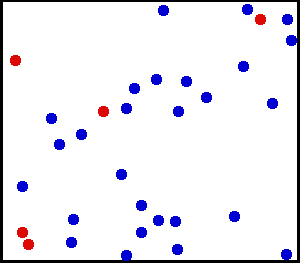## The Ideal Gas Equation

#### Learning Objective

• Apply the ideal gas law to solve problems in chemistry

#### Key Points

• An ideal gas exhibits no attractive forces between particles.
• In the ideal gas equation, both pressure and volume are directly proportional to temperature.

#### Terms

• ideal gas constantR = 8.3145 J·mol-1·K-1
• ideal gasa gas whose particles exhibit no attractive interactions whatsoever; at high temperatures and low pressures, gases behave close to ideally
• kinetic energythe energy possessed by an object because of its motion; in Kinetic Gas Theory, the kinetic energy of gas particles is dependent upon temperature only

# Kinetic Theory and Ideal Gases

All gases are modeled on the assumptions put forth by the Kinetic Theory of Matter, which assumes that all matter is made up of particles (i.e. atoms or molecules); there are spaces between these particles, and attractive forces become stronger as the particles converge. Particles are in constant, random motion, and they collide with one another and the walls of the container in which they are enclosed. Each particle has an inherent kinetic energy that is dependent upon temperature only.Translational motion of heliumReal gases do not always behave according to the ideal model under certain conditions, such as high pressure. Here, the size of helium atoms relative to their spacing is shown to scale under 1,950 atmospheres of pressure.

A gas is considered ideal if its particles are so far apart that they do not exert any attractive forces upon one another. In real life, there is no such thing as a truly ideal gas, but at high temperatures and low pressures (conditions in which individual particles will be moving very quickly and be very far apart from one another so that their interaction is almost zero), gases behave close to ideally; this is why the Ideal Gas Law is such a useful approximation.

# Ideal Gas Law Equation

The Ideal Gas Equation is given by:

$\displaystyle{PV=nRT}$

The four variables represent four different properties of a gas:

• Pressure (P), often measured in atmospheres (atm), kilopascals (kPa), or millimeters mercury/torr (mm Hg, torr)
• Volume (V), given in liters
• Number of moles of gas (n)
• Temperature of the gas (T) measured in degrees Kelvin (K)

R is the ideal gas constant, which takes on different forms depending on which units are in use. The three most common formulations of R are given by:

$\displaystyle{8.3145\frac{\text{L} \cdot \text{kPa}}{\text{K} \cdot \text{mol}}=0.0821\frac{\text{L} \cdot \text{atm}}{\text{K} \cdot \text{mol}}=62.4\frac{\text{L} \cdot \text{mm Hg}}{K \cdot \text{mol}}}$

## Example 1

A 20 L box contains a fixed amount of gas at a temperature of 300 K and 101 kPa of pressure. How many moles of gas are contained in the box?

$PV=nRT$

$\displaystyle{n=\frac{PV}{RT}=\frac{\text{(101 kPa)(20 L)}}{\text{(8.3145 }\text{L} \cdot \text{kPa} \cdot K^{-1} \cdot \text{mol}^{-1}) \cdot \text{300 K}}=\text{0.8 } \text{mol}}$

## Example 2

Calculate the number of moles of gas contained within a bouncy house with a volume of 20.63 cubic meters, a temperature of 300 Kelvin, and a pressure of 101 kPa.

$\displaystyle{PV=nRT}$

$\displaystyle{\frac{PV}{RT}=n \cdot n=\frac{101\text{ kPa} \cdot (20.63\text{ cubic meters})}{(8.3143\text{ J/mol}) \cdot K(300K)} \cdot n=835.34\text{ mols}}$

The ideal gas equation enables us to examine the relationship between the non-constant properties of ideal gases (n, P, V, T) as long as three of these properties remain fixed.

For the ideal gas equation, note that the product PV is directly proportional to T. This means that if the gas’ temperature remains constant, pressure or volume can increase as long as the complementary variable decreases; this also means that if the gas’ temperature changes, it may be due in part to a change in the variable of pressure or volume.

The ideal gas equation is a valuable tool that can give a very good approximation of gases at high temperatures and low pressures.